/

### The Dagum distribution

 Distribution name Dagum distribution Common notation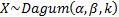Parameters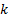= shape parameter (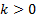)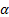= shape parameter (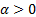)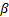= scale parameter (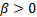) Domain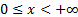Probability density function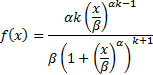Cumulative distribution function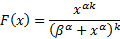Mean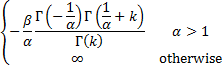Variance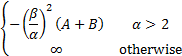where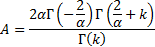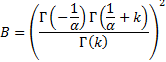Other comments Also known as the Dagum type 1 distribution. Is used in modelling income distributions. The cdf of a Dagum type 2 distribution adds a point mass at the origin and then follows a Dagum type 1 distribution over the positive halfline.   Its median is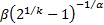and its mode is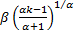.   Its non-central moments are: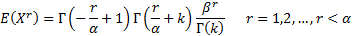Ifthen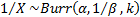.

Nematrian web functions

Functions relating to the above distribution may be accessed via the Nematrian web function library by using a DistributionName of “dagum”. Functions relating to a generalised version of this distribution including an additional location (i.e. shift) parameter may be accessed by using a DistributionName of “dagum4” ”, see also including additional shift and scale parameters. For details of other supported probability distributions see here.